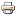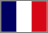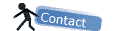# Astronomy with SalsaJ## From doppler effect to exoplanets

Learn how to find invisible bodies in other solar systems studying the spectral lines of far starsIn this exercise, we explain how an invisible planet can be unveiled using precise measurement of the velocity of its mother star. The radial-velocity method uses the fact that a star with a planet will move in its own small orbit in response to the planet's gravity. The goal is to measure variations in the speed with which the star moves toward or away from Earth. The radial velocity can be deduced from the displacement in the parent star's spectral lines due to the Doppler effect, as shown in the upper animation.

Exercice proposed by :Roger FERLET, Institut d’Astrophysique de Paris, France
Michel FAYE, lycée Louis Le Grand , Paris , France
Suzanne FAYE, Lycée Chaptal , Paris , France

Updated by :

Anne Laure Melchior, LERMA - Observatoire de Paris, France
Stefano Bertone, SyRTE - Observatoire de Paris, France

FROM DOPPLER EFFECT TO EXOPLANETS

Extract the zip file to an "exo_doppler" directory (we will call it so throughout the exercise).

Note: - ficN.fit (fit =fits = fts) are made available for the animation

- spectrN.data are made available for optical spectra analysis

1. SPECTROSCOPY

1.1 - A star emits a continuum spectrum due to nuclear reactions, with absorption lines due to the atmosphere of the star.

We shall visualize 11 spectra of a binary star, at different dates; we study the part of spectrum around Na lines: we choose Na lines because it's easy to find and also because you can have easy experiments with Na lamps or with ordinary kitchen salt.

1.2 (optional) - Experiment: The glass rod contains NaCl ; when highly heated, it produces Na light that absorbs the light from Na spectral lamp, whereas torch light looks unchanged. This phenomenon is called Na lines resonance.1.3 - Open image fic01.fit1.4 - We have 11 images.fit, at the 11 following dates

 Spectrum number Date (days) 1 0 2 0,974505 3 1,969681 4 2,944838 5 3,970746 6 4,886585 7 5,924292 8 6,963536 9 7,978645 10 8,973648 11 9,997550

The interval between two dates is roughly one day.

Each object in a binary system moves around the barycenter. So, the spectrum lines move according with time (Doppler shift).2. ANIMATED MOTION OF SPECTRUM LINES
2.1. Here is the procedure to have a global view of Doppler shift when the star moves around the barycenter of the system:

- Open SalsaJ

- Click on the Open icon :- Go to the "exo_doppler" folder (where you uncompressed the images)

- Select the 11 spectra images.fit from fic01.fit to fic11.fit: press Shift to select the 11 images.fit at once

- Open these 11 images, then

- Click on Images - Stacks - Transfer Images to Stacks

- Click again on Images / Stacks / Start animationEnjoy the Doppler shift during the rotation of the star around the barycenter of the binary system, then close.

3. MEASURE WAVELENGTH λ AND FLUX, OPTICAL SPECTRUM

Use spectrN.data

3.1. Investigation of spectrum 1: spectr1.data.

- Click on the Open icon :- Go to the "exo_doppler" folder (where you uncompressed the images)

- Select the spectr1.data and open

3.2. Flux according to wavelength : Φ= f(λ) .

You get Φ=f(λ), the brightness (flux) of the star at different wavelenghts. Notice that the scale is already set on the graph.You can observe :

-Two deep absorption lines of Na at approximately 5890 A and 5896 A;

- A smaller one (Ni I ) at 5895 A (we shall not use this one).

Open the excel file.

Press on "list" to get the list of (x,y)=(Wavelenght,Intensity) points. You now need to look for the precise wavelenght of the two intensity minima corrisponding to Na absorption lines.

You have two ways to do this :

- Look for the minimum Y (just scroll the list around 5890 A and 5896 A until you don't see Y start to grow again) and mark the correspondent X (the wavelength correspondent to the minimum intensity) in the table in the Excel file.

- (suggested) Copy data on a blank excel page (using the copy button on the graph). Select the two resulting columns and use data-order to order them by increasingly values of the B column. The first wavelenght on the ordered list is one of the searched minima, the other won't be far in the list.

BE CAREFUL!! : To look for the peak of absorption directly on the plot doesn't give you sufficient precision to get a good result in the end. You have to follow the procedure.

Now copy the two found wavelenghts λ1 and λ2 to the excel answerfile. These values will be compared to the reference values (Na lines in our laboratory) :

λ1Na = 5889,950 Ǻ                     λ2Na = 5895,924 Ǻ

The difference between reference and measured value is the Doppler shift, which will be calculated in the excel file.

3.3. Now follow the same procedure for the others 11 spectra : spectrN.data, N from 1 to 11

3.4. List of results (for correction purpose) :

 Spectrum Date λ1 (Ǻ) λ2 (Ǻ) 1 0 5890,411 5896,366 2 0.974505 5890,496 5896,511 3 1.969681 5890,491 5896,446 4 2.944838 5890,305 5896,274 5 3.970746 5890,014 5896,029 6 4.886585 5889,815 5895,8 7 5.924292 4889,642 5895,597 8 6.963536 5889,638 5895,621 9 7.978645 5889,764 5895,793 10 8.973648 5890,056 5896,042 11 9.997550 5890,318 5896,303

4. CALCULATING RADIAL VELOCITY OF THE STAR WITH DOPPLER SHIFT

4.1. You will now use the following law to deduce the radial velocity of the star (in relation to Earth) from your measures :

with

- Δλi = λi - λNai , i = 1 or 2,

- λNai reference wavelength

- λi measured wavelength of Na in the moving star spectrum.

- vrad = velocity projection of the star on the line of sight; it includes barycentre speed+ motion of the star around the system barycentre. (c = speed of light)

4.2. Once you have filled the tables for λ1 and λ2 in your Excel file, you will have the result for vrad appear in the following column. This result is an average of the value obtained using Δλ1 and Δλ2 , which improves the accuracy of your evaluation :

- Accuracy with one line : 4,2 %

- With two lines : 2%

In principle, the more lines you will use, the better accuracy you will get. This is very useful for measuring small velocities, such as stars radial velocity due to exoplanets orbiting around them.

4.3. List of results for λ1 (for correction purpose) :

 Spectrum Date λ1-λNa1 VR = c *(λ1-λNa1)/λNa1(km/s) 1 0 0.461 23.48 2 0.974505 0.546 27.81 3 1.969681 0.541 27.56 4 2.944838 0.355 18.08 5 3.970746 0.064 3.26 6 4.886585 -0.135 -6.88 7 5.924292 -0.308 -15.69 8 6.963536 -0.312 -15.89 9 7.978645 -0.186 -9.47 10 8.973648 0.106 5.40 11 9.997550 0.368 18.74

5. RADIAL VELOCITY OF THE STAR, AS A FUNCTION OF DATE

5.1. In order to obtain the radial velocity of the observed star, we will fit the measured data through a cosine.

Having filled all λ1 and λ2, you should now see a list of points in the graph below the table. Try to evaluate by eye the parameters of the fitting curve :- V0 is the offset of our data (the mean), representing the velocity of the whole system with respect to Earth ;

- W is half the amplitude of data, and it represents the radial velocity of the star ;

- T is the period ;

- Phi is the phase, -3.14 < phi < 3.14.

5.2. Once you have filled the dedicated cases, you will see your approximation appear on the graph. Try to make it better changing the parameters or go to appendix 1 to see how Excel can make it for you.

Note : Instead of Excel, you can obviously use other programs such as Regressi or Open Office Excel, which are free to download from the web.

5.3. With our model, we get (for correction purpose):

- V0 = 5.9 km/s

- A = 23.2 km/s

- Precision: 4.4%

5.4.Now that you have an evaluation for W, you should notice that, depending on the angle between the line of sight and the orbital plan of the unknown body, you would measure only a projection W of this velocity.

In this exercise, we will imagine to be perfectly aligned with the orbit of the body, so that sin(i)=1 (i being the angle between the line of sight and the perpendicular to the orbital plan). W is therefore a lower limit of the rotating velocity of the star : Vrot = W / sin(i).

6. DETERMINING THE MASS OF THE COMPANION IN BINARY SYSTEM

6.1. So, now that we have a good estimate for the radial velocity of the star, how do we calculate the mass of the unknown body orbiting around the observed star (for we still don't know if it really is a planet)?We will suppose circular orbits and use the Kepler law and some physical sense.

The 3rd Kepler's law states : T2/(SN)3 = 4 π2 / [(MS+mN) G] , where

- T is the revolution period

- MS and mN are respectively the masses of the star and the unknown object

- G = 6.67. 10- 11 uSI is Newton's gravitational constant

- O is the barycentre of the binary system (see image).

For circular orbits, we also have that : V= 2π OS/T (= W since we take sin(i) = 1)

Moreover, the barycenter laws tell us that : mN/(MS+mN) = OS/SN

It's then easy to obtain the relation (after some computations) :

2π G mN3 = V3 T (mN + MS

Since we can suppose that the star is far more massive than the unknown body, we can approximate MS+mN = MS and then easily invert the formula to obtain:

mN = V [T MN/(2π G)]1/3

Hence, using :

- the data obtained by the fit

- MS = 1.05 MSun = 1,05 * 2,0 * 1030 kg = 2,1 .1030 kg

- G = 6.67. 10- 11 uSI

and a pocket calculator, you can easily obtain the mass mN of the companion.

6.2. Results (for correction purpose) :

mN = 0.275 MS = 5,8 * 1029 kg

6.3.  Now, we can compare our result with the mass of some bodies in our solar system, to try to better identify our target :

Mass of the Earth, telluric planet : MT = 6 .1024 kg

Mass of Jupiter, giant planet : MJ = 2 .1027 kg

Mass of the Sun, main sequence star : MSun = 2,0 * 1030 kg

Since it's that larger than Jupiter, the unknown companion turns out to be a star!

It's probably a dwarf star that emits almost no visible light.

We shall also notice that we supposed sin(i) = 1, which undervalues V. That gives a lower limit on mN, which supports the idea that the unknown object is a star.

The name of the binary star you observed is HD 75767. It has been surveyed with the CORALIE spectrograph installed at the Swiss telescope at the ESO-La Silla observatory.

CONCLUSION : DISCOVERING AN EXOPLANET WITH DOPPLER SHIFT OF A STAR

The radial velocity method for detecting an exoplanet requires a very high precision which cannot be achieved within a classroom. This is why the corresponding EU-HOU exercice has been built with a binary star. The method is exactly the same, but the effect to be measured is much larger (of the order of km/s instead of m/s) and can be obtained with a single absorption line.

That's why, while this effect has been known a lot earlier, the first exoplanet was discovered only in 1995 by Mayor and Queloz at the "Observatoire de la Haute Provence" (OHP, France).(Mayor, Queloz and team, 1995)

You can see below a plot similar to the one you obtained in the exercise . It shows the radial speed of the star 51 Pegasi.Using the following data on the Pegasi system and the results of Mayor and Queloz, you are now capable of obtaining the mass of the orbiting exoplanet :

- 51-Pegasi star mass M ≈ Msun = 2,0.1030 kg

- Radial velocity of 51 Pegasi: V = 60 m/s

- Period of 51 Pegasi: T = 4,2 days.

We get a mass of 9,1.1026 kg (or 0.45 MJupiter) for the companion, called 51 Pegasi B.

This contributes to prove that 51-Pegasi B is a giant exoplanet.

IF YOU WANT TO KNOW MORE...

To better understand what kind of exoplanets it's easier (or even possible) to observe using this method, we should analyze Kepler's law.

We can roughly estimate that for an exoplanet, even a giant one, the mass mp is far lower than a star mass MS ; we can then approximate 2πGmp3 =vs3 T (mp+MS into:

m = K.V.T1/3.M2/3

, with K constant = ( 1/ 2πG )1/3.

The higher exponent above is 1 for V, and only 1/3 for T, 2/3 for M ; so, the main factor is V. A 1000 times smaller speed induces a 1000 times lower mass m, whereas a 1000 times lower period induces a 10 times lower mass m .

The rotation periods of exoplanets can vary from 3 to 3000 days, depending on orbital configuration.

We can calculate that the radial velocity variation due to a telluric planet is of the order of the 10-3-10-1 m/s, the one due to a giant planet goes from 1-100 m/s.

The Doppler method to detect exoplanets needs thousands of lines in the spectrum of the star to reach a m/s accuracy: so it works well for giant exoplanets quite near to their star (the period of revolution T is proportional to the distance from the star).

We cannot yet (2011) detect a Doppler shift due to a telluric exoplanet : radial velocity is still too small (but we're getting closer)!!